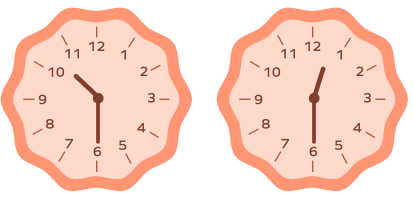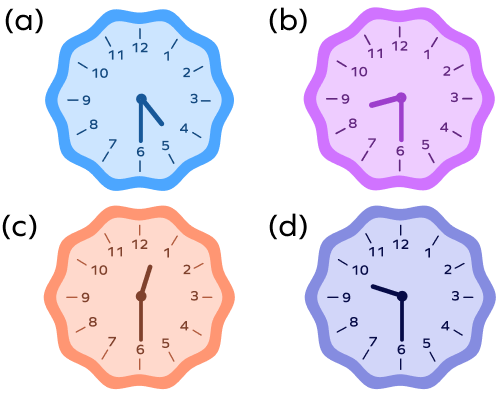# Half Past the Hour

Home » Math-Vocabluary » Half Past the Hour

## Half Past the Hour: Introduction

Looking at a clock, we can say that one hour equals 60 minutes. When we divide an hour into half, it equals 30 minutes. When the hands of a clock complete an entire hour, the larger of the two hands of the clock, points at 12.

Also known as the minute hand, it points at 6 if the clock completes 30 minutes. This is when we mention the time as half past the hour.

## What Is Half Past the Hour?

To answer this we first need to understand the definition of half past.

What does half past mean? Half past means that half of an hour has passed. Since an hour equals 60 minutes, half of an hour is the same as 30 minutes. So half past the hour also implies 30 minutes past the hour

Let’s look at some half past the hour examples to understand this better.

For example, When we say half past 3, we mean that half hour i.e 30 minutes past 3.

The time is half past the hour if the larger hand of the clock points at 6.

For example, in the above image, the shorter hand of the clock, or the hour hand, is pointing between 7 and 8. At the same time, the minute hand is pointing at 6.

Thus, we can say that the time is half past the hour 7. We can say this because the hour hand has gone past 7 but not reached 8, which is why it is halfway between 7 and 8.

## Where Is Half Past on the Clock?

Every time the minute hand of a clock reaches 12, a new hour begins. When the minute hand completes a half turn, we can say that the time is half past the hour. So in a half past clock , the minute hand will always point downwards at 6. It indicates that 30 minutes have passed since the new hour began. Whereas the hour hand will point halfway between the two numbers representing hours, indicating that a half hour has passed.

In the above image, the time is half past 1. Notice how the minute hand is pointing at 6 and the hour hand is between 1 and 2. We can tell that the time is half past the hour because the minute hand can be seen pointing at 6. Also, the hour hand will be halfway between any two numbers on the clock.

## How Do You Express Half Past the Hour?

We have understood the meaning of half past the hour and what it means. To express the time, we can write it down by mentioning the hour and minutes. For example, half past 2 o’clock means that the time is 2:30. Similarly, half past 12 means that the time is 12:30.

### How Do You Show Half Past 2?

The following image depicts a half past 2 on clock.

To show the time is half past 2, we will draw the minute hand pointing at 6 and the hour hand should have crossed 2 so it would be midway between 2 and 3.

## Reading Half Past the Hour on an Analog Clock

We can only tell what time a clock is showing by understanding its different parts. The longer hand on a clock is the minute hand. Every hour, it makes one complete turn from 12 to 12, which totals 60 minutes. The shorter hand of the clock is the hour hand.

Every hour, the hand moves from one number to the next one. During the day, the hour hand makes two whole turns on a clock since there are 24 hours in a day. We can look at the shorter hand if we want to know what hour it is. If it is not directly pointing at a number, then we can say it has passed that hour by certain minutes. The minute hand will tell us how many minutes past the hour it is.

When the time is half past the hour on a clock, the minute hand can be seen pointing at the number 6, which indicates that 30 minutes have passed since the hour began.

## Teaching Half Past the Hour

These steps will help you to understand how to read half past the hour on a clock.

• If the clock’s long hand or the minute hand is pointing at 6, then the time is half past the hour.
• Next, look for the shorter hand of the clock and the two numbers between which it lies.
• The time is half past the smaller of the two numbers. The only exception is when the hour hand is between 12 and 1. At that time, the time is half past 12.

Take, for example, let’s look at this clock:

Following the steps mentioned above, since the minute hand is at 6, we know that the time is half past in the clock. Further, we can see that the hour hand is between 8 and 9. 8 is the smaller of the two numbers, so the time is half past 8. We can also mention the time as 30 minutes past 8 or 8:30.

## Conclusion

Learning how to look at a clock and tell the time can be fun. Half past the hour is a common way to speak the time, so it is important to learn what it means.

## Solved Examples

1. Take a look at the image below. What is common about the time in each one of them?

Solution: Each of the clocks above displays an example of half past the hour since the minute hand can be seen pointing at 6, and the hour hand lies between two consecutive numbers.

2. Which of the following clock faces shows the time half past 12?

Solution: The third clock (c) shows the correct display for half past 12 since the clock’s minute hand is at 6, and the hour hand lies between 12 and 1. The hour hand has crossed 12 but hasn’t reached 1. So it’s past 12.

3. Take a look at the clock shown below. What time does it depict?

Solution: As we can see, the hour hand lies between 5 and 6, while the minute hand lies on 6. Thus, the time is half past 5.

4. Does the clock below show half past 8?

Solution: Since the minute hand does not lie on 6 exactly, we cannot say that the time is half past the hour.

5. At half past the hour, how many minutes remain before the minute hand of the clock completes a whole round to complete the hour?

Solution: We know that at half past the hour, the clock’s minute hand lies at 6, which is the equivalent of 30 minutes. Since one hour is 60 minutes, the minute hand must move another 30 minutes to complete the hour.

## Practice Problems

1

### Mr. A is attempting an exam. He has 3 hours to complete all the questions. The exam began at 10:00 in the morning, and now, he has half an hour to go before the end of the exam. What is the time right now?

1:30 p.m.
12:30 p.m.
2:00 p.m.
11:30 a.m.
CorrectIncorrect
Given that the exam began at 10:00 a.m., we know that only half an hour remains before the end of the exam. Thus, the total time elapsed since the exam started is 2:30 hours. The time at present is 12:30 p.m.
2

### Which of the following two clocks represents 10:30?First
Second
Both
None
CorrectIncorrect
The time 10:30 means half past 10, which means that the minute hand must be at 6, and the hour hand must lie between 10 and 11.
3

### Which of the following alarm clocks depicts the time 12:30?A
B
C
D
CorrectIncorrect
Identifying a clock reading half past 12
4

### If Jess started running in a park at ten minutes past 9, how many minutes must she run if she wanted to end her run at half past 9?

20 minutes
40 minutes
10 minutes
60 minutes
CorrectIncorrect
At ten minutes past 9, the time is 9:10. To complete her run at 9:30, Jess must run the differential time, which is 20 minutes.
5

### Take a look at the alarm clock below. Although it does not depict the hands of the clock, which of the following options is the best depiction of the time on the watch?30 minutes to 5
Quarter past 5
Quarter to 5
Half past 5
CorrectIncorrect
Correct answer is: Half past 5
The time on the clock is 5:30, which is a true depiction of half past 5 when 30 minutes have passed since the beginning of the hour.